Next: Exercises Up: Quantum Dynamics Previous: Gauge Transformations in Electromagnetism

# Flux Quantization and the Aharonov-Bohm Effect

Consider a situation in which the electric and magnetic fields are non-time-varying. In this case, Equation (3.89) becomes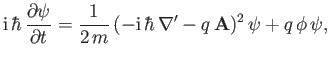(3.103)

where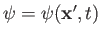,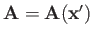, and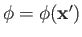. The previous equation has the formal solution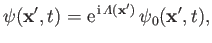(3.104)

where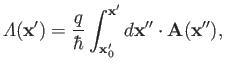(3.105)

and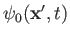is a solution of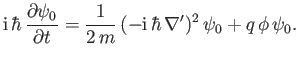(3.106)

Here,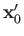is an arbitrary fixed point. However, the solution (3.104) only makes sense in a region in which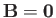. To see this, let us imagine calculating the phase factor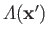by evaluating the line integral specified in Equation (3.105) along two different paths, labelled 1 and 2, that join the pointsand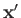. We find that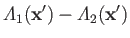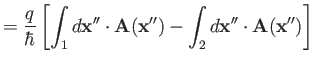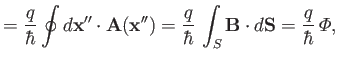(3.107)

where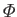is the magnetic flux passing through a surface spanning the two paths. Here, we have made use of the curl theorem , as well as Equation (3.73). Thus, the phase factoris only independent of the choice of path in the line integral when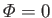. Such independence is required if we insist that the wavefunction be single valued.

Suppose that a charged particle moves in a magnetic-field-free region that is not simply connected, but surrounds a hole through which the magnetic fluxpasses. Upon completing a circuit around the hole, the particle's wavefunction is multiplied by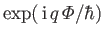. The requirement that the wavefunction be single-valued, and, hence, that the multiplication factor be unity, implies that the enclosed magnetic flux is quantized. In fact.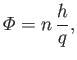(3.108)

where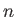is an integer. This effect is known as flux quantization.

A situation like that just described arises in the motion of electrons in a superconducting ring through which a magnetic field passes. Incidentally, superconductors contain no internal magnetic fields because they expel magnetic flux--this behavior is called the Meissner effect . Experimentally, the magnetic flux passing through a superconducting ring is found to satisfy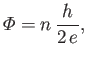(3.109)

whereis an integer, and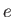the magnitude of the electron charge [27,33]. This result is consistent with our present understanding of the phenomenon of superconductivity, according to which the fundamental charge carriers are correlated electron pairs known as Cooper pairs .

Consider an electron interference experiment in which a small solenoid containing magnetic flux is placed directly behind the slits of a two-slit interference apparatus. The interference pattern at the screen is due to the superposition of two parts of the wavefunction,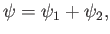(3.110)

where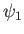and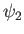are the wavefunctions due to electrons that originate from the source, pass through the first and second slits, respectively, and then strike the same point on the screen. When the solenoid is energized an additional phase shift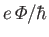is introduced betweenand, whereis the net flux passing through the solenoid. In other words, the magnetic field internal to the solenoid affects the interference pattern seen on the screen, despite the fact that neither electron beam ever directly experiences a magnetic field (because the field is internal to the solenoid, and the two interfering beams are assumed to pass on either side of the solenoid.) This phenomena is known as the Aharonov-Bohm effect , and has been observed experimentally .Next: Exercises Up: Quantum Dynamics Previous: Gauge Transformations in Electromagnetism
Richard Fitzpatrick 2016-01-22